• ## 循环矩阵傅里叶对角化

万次阅读 多人点赞 2016-03-15 14:10:30
“任意循环矩阵可以被傅里叶变换矩阵对角化”这个概念常常出现在论文中，本文对其做简单解释。

All circulant matrices are made diagonal by the Discrete Fourier Transform (DFT), regardless of the generating vector x.
任意循环矩阵可以被傅里叶变换矩阵对角化。

文献中，一般用如下方式表达这一概念：
$X=C(x)=F \cdot diag(\hat{x}) \cdot F^H$
其中$X$是循环矩阵，$\hat{x}$是原向量$x$的傅里叶变换，$F$是傅里叶变换矩阵，上标H表示共轭转置：$X^H=(X^{*})^T$。
换句话说，$X$相似于对角阵，$X$的特征值是$\hat x$的元素。
另一方面，如果一个矩阵能够表示成两个傅里叶矩阵夹一个对角阵的乘积形式，则它是一个循环矩阵。其生成向量是对角元素的傅里叶逆变换：
$F \cdot diag(y) \cdot F^H = C(\mathcal{F}^{-1}(y))$
这个公式初看疑问很多，以下一一讨论。
$X$是什么？
$X$是由原向量$x$生成的循环矩阵。以矩阵尺寸$K=4$为例。
$X=C(x)=\begin{bmatrix} x_1 & x_2 & x_3 & x_4 \\ x_4 & x_1 & x_2 & x_3 \\ x_3 & x_4 & x_1 & x_2 \\ x_2 & x_3 & x_4 & x_1 \\ \end{bmatrix}$
$F$ 是什么？
$F$是离散傅里叶矩阵（DFT matrix），可以用一个复数$\omega = e^{-2\pi i/K}$表示，其中$K$为方阵$F$的尺寸。以$K=4$为例。
$F=\frac{1}{\sqrt{K}} \begin{bmatrix} 1 & 1 & 1 & 1 \\ 1 & \omega & \omega^2 & \omega^3 \\ 1 & \omega^2 & \omega ^4 & \omega^6 \\ 1 & \omega^3 & \omega^6 & \omega^9 \end{bmatrix}$
把$\omega$想象成一个角度为$2\pi/K$的向量，这个矩阵的每一行是这个向量在不断旋转。从上到下，旋转速度越来越快。
之所以称为DFT matrix，是因为一个信号的DFT变换可以用此矩阵的乘积获得：
$\hat{x}=DFT(x)=\sqrt{K}\cdot F \cdot x$
反傅里叶变换也可以通过类似手段得到：
$x=\frac{1}{\sqrt{K}}F^{-1}\hat{x}$
傅里叶矩阵有许多性质：

是对称矩阵，观察$\omega$的规律即可知；
满足$F^HF=FF^H=I$，也就是说它是个酉矩阵（unitary）。可以通过将$F^{H}$展开成$\omega^{H}$验证。

注意：$F$是常数，可以提前计算好，和要处理的$x$无关。
对角化怎么理解？
把原公式两边乘以逆矩阵：
$F^{-1} \cdot X \cdot (F^H)^{-1}=diag(\hat{x})$
利用前述酉矩阵性质：
$左边=F^{H}XF=diag(\hat{x})$
也就是说，矩阵$X$通过相似变换$F$变成对角阵$diag(\hat{x})$，即对循环矩阵$X$进行对角化。
另外，$F^HXF$是矩阵$X$的2D DFT变换。即傅里叶变换可以把循环矩阵对角化。
怎么证明？
可以用构造特征值和特征向量的方法证明（参看这篇论文1的3.1节），此处简单描述。
考察待证明等式的第k列：
$X\cdot f_k=\hat x_k\cdot f_k$
其中$f_k$表示DFT矩阵的第k列，$\hat x_k$表示傅里叶变换的第k个元素。等价于求证：$f_k$和$\hat x_k$是$X$的一对特征向量和特征值。
左边向量的第i个元素为：$left_i = \left[ x^i, f_k \right]$。$x_i$表示把生成向量$x$向右移动i位，$[]$表示内积。
内积只和两个向量的相对位移有关，所以可以把$f_k$向左移动i位：$left_i=\left[ x, f_k^{-i}\right]$。
DFT矩阵列的移位可以通过数乘$\omega$的幂实现：$f_k^i=f_0\cdot \omega^{ik}$。

举例：$K=3$，
$F=\frac{1}{\sqrt{K}} \begin{bmatrix} 1 & 1 & 1\\ 1 & \omega & \omega^2 \\ 1 & \omega^2 & \omega ^4 \end{bmatrix}$

利用$\omega^N=1$.
$f_1\cdot \omega =[1,\omega,\omega^2]\cdot \omega = [\omega, \omega^2,\omega^3] = [\omega, \omega^2, 1]=f_1^{-1}$

$f_1\cdot \omega^2 =[1,\omega,\omega^2]\cdot \omega^2 = [\omega^2, \omega^3,\omega^4] = [\omega^2, 1, \omega]=f_1^{-2}$

于是有：
$left_i= \left [x, f_k \cdot \omega^{ik}\right ]=\left [x, f_k\right ] \cdot \omega^{ik}$
右边的$\hat x = F\cdot x $，考虑到$F$的第k行和第k列相同，$\hat x_k=[f_k,x]$。另外$f_k$的第i个元素为$\omega^{ik}$：
$right_i = \hat x_k\cdot f_{ki}=[f_k^*,x]\cdot \omega^{ki}$
对任意k列的第i个元素有：$left_i=right_i$
更多性质
利用对角化，能推导出循环矩阵的许多性质。
转置
循环矩阵的转置也是一个循环矩阵（可以查看循环矩阵各元素排列证明），其特征值和原特征值共轭。
$X^T=F \cdot diag((\hat{x})^*) \cdot F^H$
可以通过如下方式证明：
$X^T=(F^H)^T\cdot diag(\hat x) F^T$
由于$F$是对称酉矩阵，且已知$X$是实矩阵：
$X^T=F^*\cdot diag(\hat x) F=\left( F^*\cdot diag(\hat x) F\right)^*=F\cdot diag((\hat x)^*) \cdot F^H$
如果原生成向量$x$是对称向量（例如[1,2,3,4,3,2]），则其傅里叶变换为实数，则：
$X^T=C\left( \mathcal F^{-1}(\hat x)^* \right)=C(x)$
卷积
循环矩阵乘向量等价于生成向量的逆序和该向量卷积，可进一步转化为福利也变化相乘。
注意卷积本身即包含逆序操作，另外利用了信号与系统中经典的“时域卷积，频域相乘”。
$\mathcal{F}(Xy) = \mathcal{F}(C(x)y)=\mathcal{F}(\bar x*y)=\mathcal{F}^*(x)\odot \mathcal{F}(y)$
其中$\bar x$表示把$x$的元素倒序排列。星号表示共轭。
相乘
设$C,B$为循环矩阵，其乘积的特征值等于特征值的乘积：
$AB = F\cdot diag(\hat a) \cdot F^H \cdot F \cdot diag(\hat b) \cdot F^H$
$= F\cdot diag(\hat a) \cdot diag(\hat b) \cdot F^H=F\cdot diag(\hat a \odot \hat b) \cdot F^H$
$=C\left( \mathcal F^{-1}(\hat a \odot \hat b)\right)$
乘积也是循环矩阵，其生成向量是原生成向量对位相乘的傅里叶逆变换。
相加
和的特征值等于特征值的和：
$A+B = F\cdot diag(\hat a) \cdot F^H + F \cdot diag(\hat b) \cdot F^H=F\cdot diag(\hat a +\hat b) \cdot F^H$
$=C\left( \mathcal F^{-1} (\hat a +\hat b)\right)=C\left( \mathcal F^{-1} (\hat a )+F^{-1} (\hat b )\right)=C\left( a+b\right)$
和也是循环矩阵，其生成向量是原生成向量的和。
求逆
循环矩阵的逆，等价于将其特征值求逆。
$X^{-1}=F\cdot diag(\hat{x})^{-1}F^H=C\left( \mathcal F^{-1}(diag(\hat{x})^{-1}) \right)$
对角阵求逆等价于对角元素求逆。以下证明：
$F\cdot diag(\hat{x})^{-1}\cdot F^H \cdot F\cdot diag(\hat{x}) \cdot F^H$
$=F\cdot diag(\hat{x})^{-1}\cdot diag(\hat{x}) \cdot F^H=F\cdot F^H=I$
逆也是循环矩阵
有什么用？
该性质可以将循环矩阵的许多运算转换成更简单的运算。例如：
$X^HX=F \cdot diag(\hat{x} \odot \hat{x}^*)\cdot F^H=C\left( \mathcal F^{-1}(\hat{x} \odot \hat{x}^*) \right)$
原始计算量：两个方阵相乘（$O(K^3)$）
转化后的计算量：反向傅里叶($K\log K$)+向量点乘（$K$）。
CV的许多算法中，都利用了这些性质提高运算速度，例如2015年TPAMI的这篇高速跟踪KCF方法2。
二维情况
以上探讨的都是原始信号为一维的情况。以下证明二维情况下的$F^HXF=diag(\hat x)$，推导方法和一维类似。
$x$是二维生成矩阵，尺寸$N\times N$。
$X$是一个$N^2\times N^2$的分块循环矩阵，其uv块记为$x^{uv}$，表示$x$下移u行，右移v列。
$F$是$N^2\times N^2$的二维DFT矩阵，其第uv块记为$f_{uv}=\left\{ \omega^{ui+vj} \right\} _{ij}$。

举例：N=3 $f_{01}=\begin{bmatrix} 1 & \omega & \omega^2 \\ 1 & \omega & \omega^2 \\ 1 & \omega & \omega^2\end{bmatrix}, f_{11}=\begin{bmatrix} 1 & \omega & \omega^2 \\ \omega & \omega^2 & \omega^3 \\ \omega^2 & \omega^3 & \omega^4\end{bmatrix}\\$

需要验证的共有$N\times N$个等式，其中第$uv$个为：
$[X, f_{uv}]=\hat x_{uv}\cdot f_{uv}$
其中$[X, f_{uv}]$表示把$x^{uv}$分别和$f_{uv}$做点乘，结果矩阵元素求和。
这个等式的第ij元素为：
$[x^{ij},f_{uv}]=\hat x_{uv} \cdot \omega^{ui+vj}$
再次利用两个性质：1) 点乘只和两个矩阵相对位移有关，2) $f_{uv}$的位移可以用乘$\omega$幂实现：
$left_{ij}=[x,f_{uv}^{-i-j}]=[x,f_{uv}]\cdot \omega^{ui+vj}=\hat x_{uv} \cdot \omega^{ui+vj}=right_{ij}$
代码
以下matlab代码验证上述性质。需要注意的是，matlab中的dftmatx函数给出的结果和本文定义略有不同，需做一简单转换。另外，matlab中的撇号表示共轭转置，transpose为转置函数，conj为共轭函数。
clear;clc;close all;

% 1. diagnolize
K = 5;      % dimension of problem

x_base = rand(1,K);     % generator vector
X = zeros(K,K);         % circulant matrix
for k=1:K
X(k,:) = circshift(x_base, [0 k-1]);
end

x_hat = fft(x_base);    % DFT

F = transpose(dftmtx(K))/sqrt(K);       % the " ' " in matlab is transpose + conjugation

X2 = F*diag(x_hat)*F';

display(X);
display(real(X2));

% 2. fast compute correlation
C = X'*X;
C2 = (x_hat.*conj(x_hat))*conj(F)/sqrt(K);

display(C);
display(C2);


Gray, Robert M. Toeplitz and circulant matrices: A review. now publishers inc, 2006. ↩︎

Henriques, João F., et al. “High-speed tracking with kernelized correlation filters.” Pattern Analysis and Machine Intelligence, IEEE Transactions on 37.3 (2015): 583-596. ↩︎


展开全文傅里叶变换
• Note：PCA主成分分析用到实对称的相似对角化。1.对角阵概念2.矩阵对角阵相似的条件3.一般矩阵的相似对角化4.实对称矩阵的相似对角化5.协方差矩阵的相似对角化(end)...
Note：PCA主成分分析用到实对称阵的相似对角化，用个文章复习一下相关概念和计算过程。1.对角矩阵如果一个矩阵满足如下条件，则它就是一个对角阵：（1）是一个方阵（2）只有对角线元素是非零元素形状如：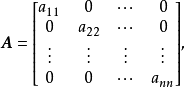2.数量矩阵如果一个矩阵满足如下条件，则它就是一个数量矩阵：（1）是一个方阵（2）只有主对角线上元素是非零元素（3）主对角线上元素都相等！也就是：对角线元素都相等的对角矩阵是数量矩阵。可知单位矩阵E是数量矩阵的特殊情况3.正对角阵只有正对角线上元素为非零值时，称为正对角阵，如下所示：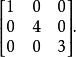4.反对角阵只有副对角线上元素为非零值时，称为反对角阵，如下所示：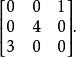5.线性相关、线性无关在下面理解矩阵与对角阵相似的过程中，涉及到了线性无关，记录下。线性相关：在一组数据中，有一个或者多个量可以被其余量表示线性无关：在一组数据中，没有一个量可以被其余量表示6.矩阵与对角阵相似的条件如果一个矩阵A满足如下条件，则此矩阵就可以说是对角矩阵相似：（1）A是一个方阵，因为对角阵是方阵（2）矩阵A有n个线性无关的特征向量如何用计算方阵A的特征值的方法来判断方阵A 是否与对角阵相似？答，步骤如下：（1）先求出方阵A的所有特征值（2）如果所有特征值互异，则方阵与对角阵相似即：如果n阶方阵A有n个互异的特征值，则方阵A与对角阵相似7.一般矩阵的相似对角化如果方阵A与对角阵相似，则一定存在一个可逆矩阵P，按照下面公式求出方阵A的相似对角矩阵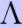：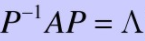求方阵A相似对角阵的步骤：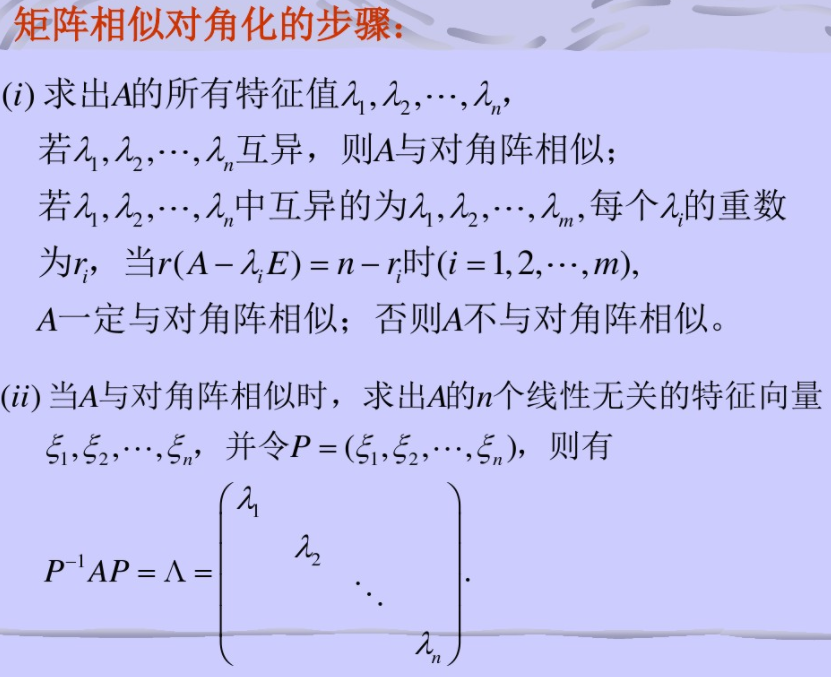8.一般矩阵对角化的练习题此例题来自：点我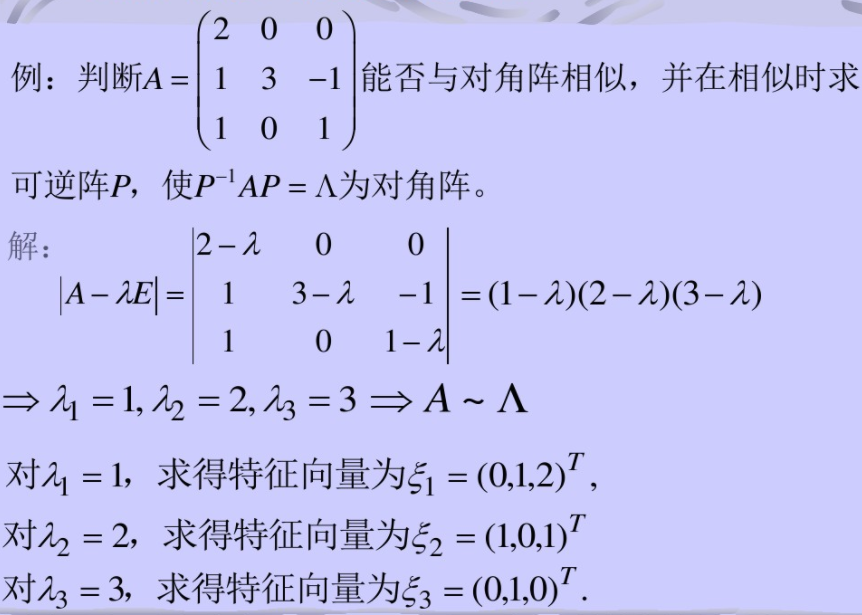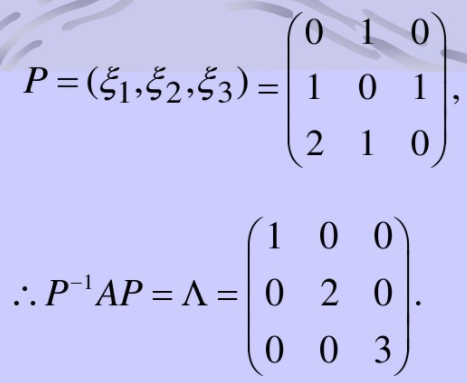9.实对称矩阵的相似对角化方法：可以用正交阵将实对称矩阵A化为对角阵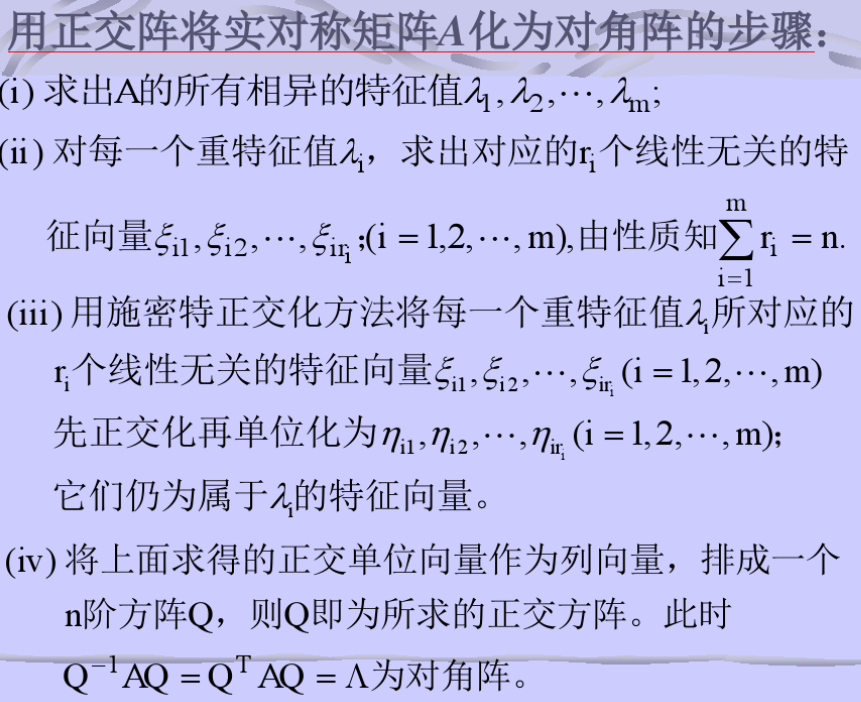10.实对称矩阵的相似对角化的练习题此例题来自：点我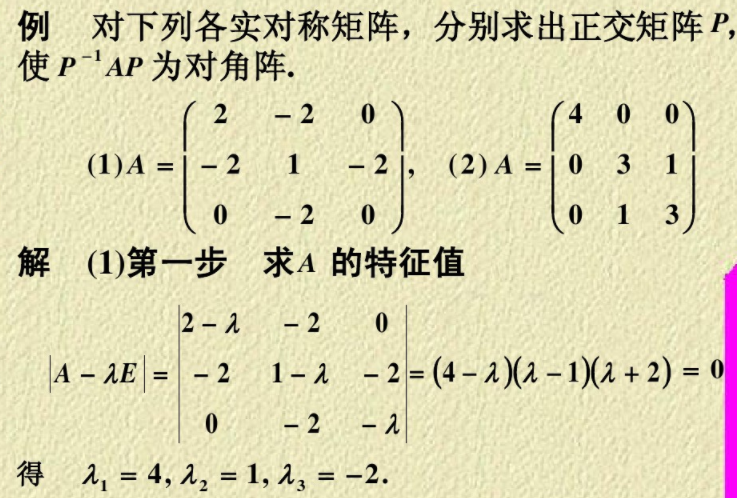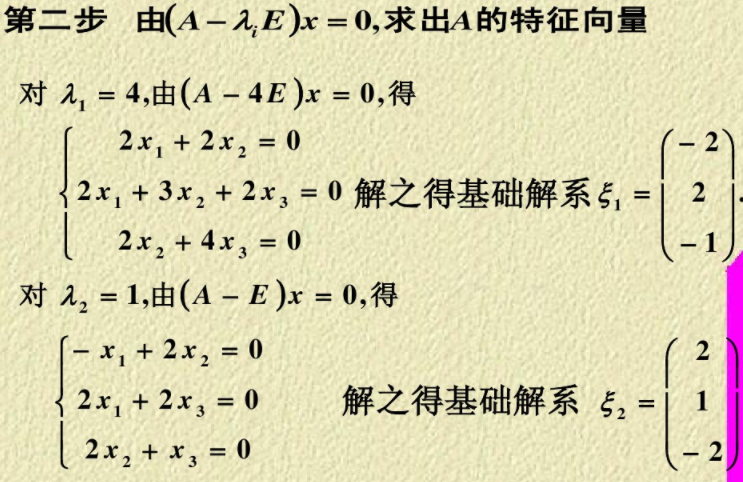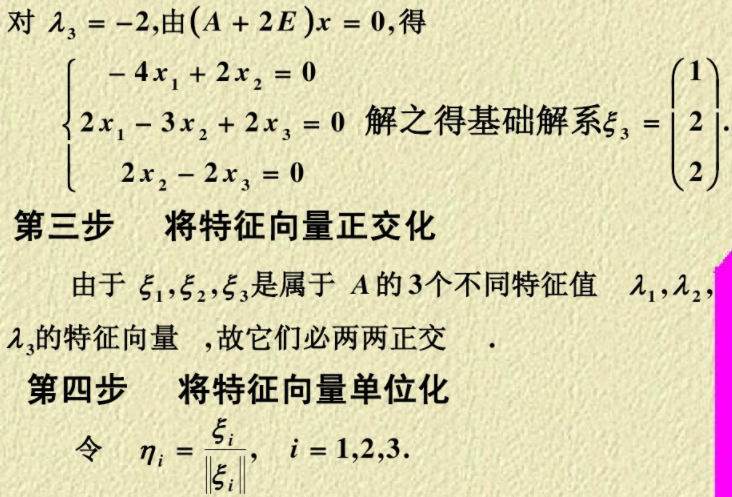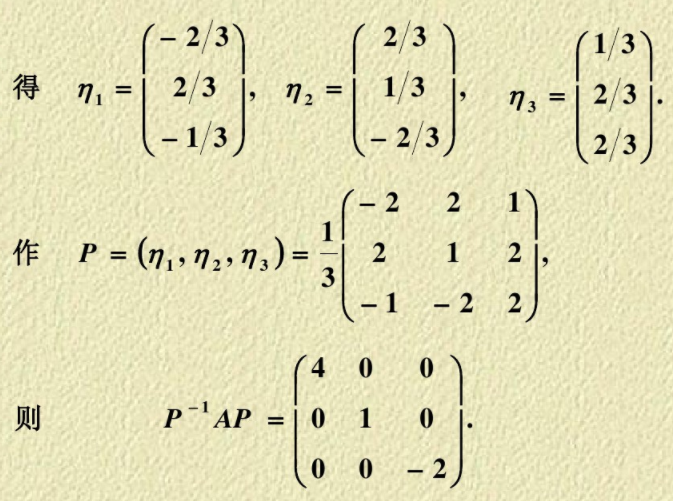11.协方差矩阵的相似对角化因为协方差矩阵是实对称矩阵，所以协方差矩阵的对角阵求解方法 可以按照 实对称矩阵的对角阵求解方法来计算，如上所示。(end)
展开全文• 026 矩阵对角化
026 矩阵对角化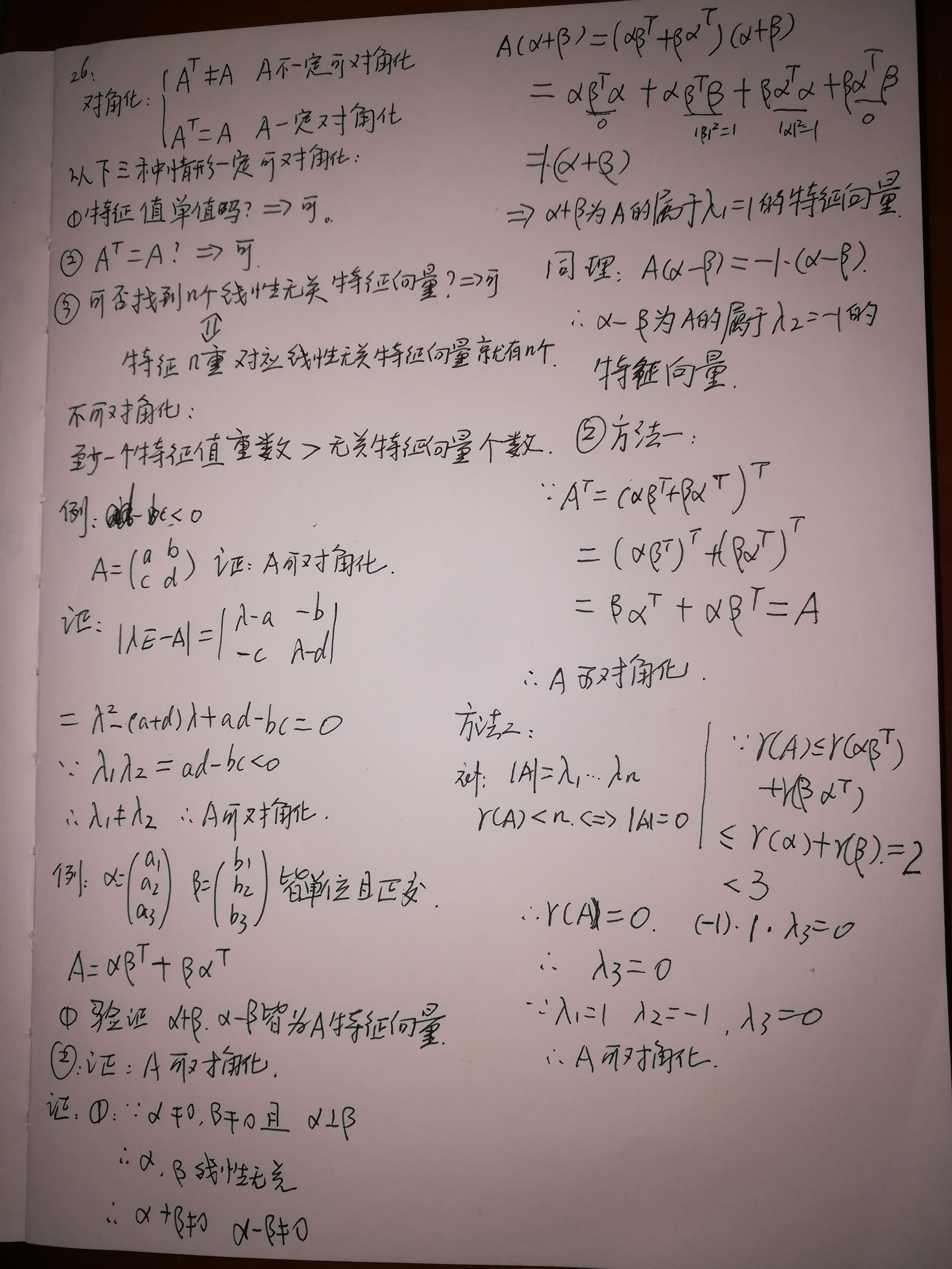展开全文矩阵 特征值 特征向量
• 本文从矩阵为什么要对角化讲到什么不能对角化，解释不能对角化是什么意思可参看视频，主要思想表述如下： 矩阵相似对角化与不能对角化的解释 矩阵相似对角化的进一步理解，几何加本质 对一般矩阵的研究转化...
矩阵不相似于对角阵的几何解释
本文从矩阵为什么要对角化讲到为什么不能对角化，解释不能对角化是什么意思可参看视频，主要思想表述如下：
矩阵相似对角化与不能对角化的解释

矩阵相似对角化的进一步理解，几何加本质

对一般矩阵的研究转化为幂零矩阵的研究，
把矩阵当作变换，研究
特征方向不够多是因为映射的幂零性造成的
若当块幂零阵把一个空间所有向量拉到一个方向，就是特征方向


展开全文机器学习 人工智能 计算机视觉 深度学习 matlab
• 2 ，对角矩阵 ： 定义 ： 1 ，主对角线的元素不 0 2 ，其他元素都 0 例如 ： 3 ，正定矩阵 ： 定义 ： 1 ，可以让非零实向量乘以他自己的转置 > 0 ，这样的矩阵叫正定矩阵 2 ，理解 ： 把它的方向正过来 ...线性代数
• 其中A为对角矩阵，P单位正交。对于一般的方阵而言，不一定能对角，对于对称矩阵而言，必能对角，而且对于正定矩阵有λ\lambdaλi>0。 设A=[λ1λ2λn]\left[\begin{matrix} \lambda_1& &\\&\...
• ## 矩阵的对角化

千次阅读 2015-03-29 10:16:53
矩阵对角化 讲解矩阵对角化之前，先了解下相似矩阵。 相似矩阵的定义：A、B都是n阶矩阵，若存在可逆矩阵P,使得P-1AP = B，(注意全文中所有的P-1=P的逆矩阵)则定义矩阵B是矩阵A的相似矩阵或称矩阵A与矩阵B...
• 对角：若方阵A相似于对角矩阵，即存在可逆矩阵P和对角矩阵D，有，则称A可对角。 可对角的充要条件： n*n阶矩阵A可对角的充分必要条件是矩阵A有n个线性无关的特征向量。 充分性证明： 设A的n个线性无关的...
• ## 线性代数笔记8：矩阵的对角化

万次阅读 多人点赞 2018-04-02 21:33:36
定义一：若存在可逆矩阵SSS，使得S−1ASS−1ASS^{-1}AS为对角矩阵，则称为矩阵AAA是可对角的（diagonalized）。 设n×nn×nn\times n矩阵有nnn个线性无关的特征向量x1,...,xnx1,...,xnx_1,...,x_n，令S=(x1,...,...线性代数
• 什么实对称矩阵的相似...对称矩阵也可以用一般的由特征向量组成的非奇异对角化，只不过它有特殊的性质（对称），因此我们就可以考虑特殊的对角化，也就是正交相似对角化。这么做有好处：正交矩阵的逆矩阵很容...
• - [矩阵对角化](#矩阵对角化) - [SVD分解](#svd分解) - [参考链接](#参考链接) 矩阵对角化 矩阵的相似 设 A\boldsymbol{A}A、 B\boldsymbol{B}B 两个nnn阶矩阵，若存在可逆矩阵 P\boldsymbol{P}P，使得...线性代数 SVD
• 线性变换A‾\underline{A}A​可对角化   ⟺  \iff⟺ 存在一组基，使得线性变换A‾\underline{A}A​在这组基下的矩阵为对角阵 线性变换A‾\underline{A}A​在各个基下的矩阵构成AAA的等价类，所以： 存在一组基，...
• ## 矩阵对角化

千次阅读 2015-11-02 12:15:57数学
• 总结：对于任意方阵，如果没有重根，矩阵总是可以对角。麻烦的是重根问题 如果有重根，那么需要验证所谓几何重数，与代数重数相等。...由矩阵对角化的推广就引出了奇异值分解和Jordan标准型。 ...
• ## 相似矩阵、矩阵的相似对角化

万次阅读 多人点赞 2016-10-19 19:12:47
特殊的，如果A∼Λ,Λ是对角矩阵A \sim \Lambda, \Lambda 是对角矩阵, 则称A可以相似对角。Λ\Lambda是相似标准形。矩阵可相似对角的充要条件 n阶矩阵A可对角 ⟺\Longleftrightarrow A有n个线性无关的特征向线性代数
• 矩阵的对角、SVD分解及应用矩阵的对角、SVD分解及应用矩阵运算的总结矩阵对角化SVD分解张量(tensor)转置(transpose) 矩阵的对角、SVD分解及应用 许多数学对象可以通过将它们分解成多个组成部分或者找到它们的...SVD
• 矩阵对角化：AS = S（讲AS展开可以推导出这个公式） 上式两边的左边同时乘以S-1，得出S-1AS = 。这就是方阵的对角公式 上式两边的右边同时乘以S-1，得出A = SS-1，这就是矩阵的句对话分解。   如果A的特征值...
• ## 矩阵可对角化条件

千次阅读 2019-08-31 16:21:28
除了对角矩阵元素的排列次序可变动， A A A 的相似标准形是唯一的 定理2 设 λ 1 , λ 2 \lambda_1,\lambda_2 λ 1 ​ , λ 2 ​ 是数域 K K K 上的 n n n 级矩阵 A A A 得到不同特征值， α 1 , α 2 , . . ....
• R语言产生各种类型的矩阵矩阵运算R语言产生一般的矩阵R语言产生单位R语言产生次对角阵R语言矩阵的常见运算 R语言产生一般的矩阵 # 依行排列，产生3行5列的矩阵 A = matrix(c(1:15),3,5,byrow=T) R语言产生单位...线性代数 数据挖掘
• 对角的两个小定理小唠嗑定理1:矩阵AAA可对角的充要条件是AAA有nnn个线性无关的特征向量小定理1:矩阵AAA可对角等价于矩阵AAA与一个对角矩阵相似定理2:任意nnn级实对称矩阵AAA都正交相似于一个对角矩阵小定理：...线性代数 抽象代数 机器学习
•矩阵 二次型
• 　最终得到了S和一个以特征值对角线的对角矩阵的乘积，这个对角矩阵就是特征值矩阵，用Λ表示： 　没有人关心线性相关的特征向量，上式有意义的前提是S由n个线性无关的特征向量组成，这意味着S可逆，等式两侧...
• 如果一个矩阵时一个上三角、下三角或者对角矩阵，这个带来很大的方便。但是往往很多矩阵都不是对角矩阵，本文就来介绍如何使用特征值和特征向量把一个矩阵变成对角矩阵！ 1.对角 我们假设一个n*n的矩阵有n个线性...特征向量
• numpy 操作矩阵的意义 1.可以理解矩阵运算，多维运算 2.可以用于理解tensorflow，pytorch的tensor张量运算，二维张量就是矩阵 例如新建一个矩阵 a = np.arange(1,10).reshape(3,-1) 上下三角矩阵 a = np.arange(1,...python
• 原来，实对称矩阵对角化解决解析几何中二次曲面是何类型而提出的，因为二次曲面的方程可以写成(x,y,z)A(xyz)(x,y,z)A\begin{pmatrix}x\\y\\z\end{pmatrix}(x,y,z)A⎝⎛​xyz​⎠⎞​的形式，而这里的A就是一个实......